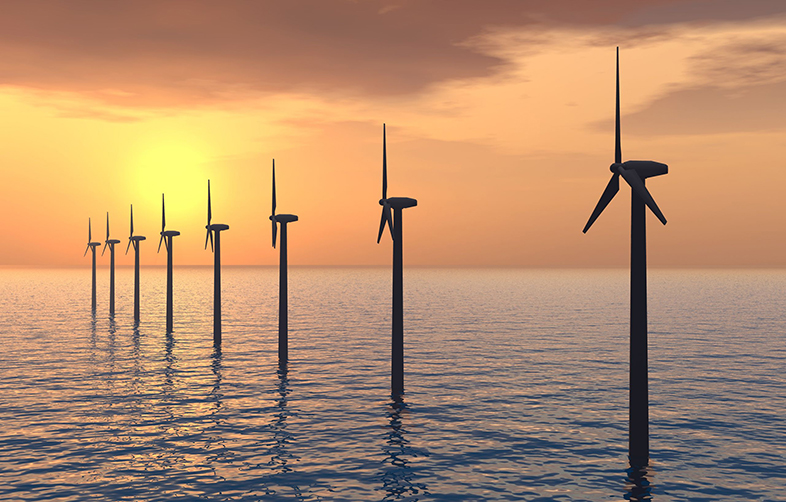Can renewable energy sources power the world?

Start this free course now. Just create an account and sign in. Enrol and complete the course for a free statement of participation or digital badge if available.

Free course

# 5 Stored energy and available power

## Box 1: Greek letters

In science and engineering it is common to use Greek letters for certain quantities and we are going to introduce two here:

ρ (pronounced "rho") is used to represent the density of a substance, that is the mass of it that appears in a given volume of that substance. In SI units this is expressed in kilogrammes per metre cubed, kg m-3.

η (pronounced "eta") is used to represent the efficiency of the turbine, that is the fraction of the energy of the flowing water that is converted into electrical energy by the turbine. This is expressed as a number between 0 and 1, and may often be stated as a percentage. For example, an efficiency of 85% is 0.85. (Remember that the "%" symbol means /100, so 85% = 85/100 = 0.85)

Water held at a height represents gravitational potential energy. If m kilograms are raised through H metres, the stored potential energy in joules is given by the following simple equation in Figure 6.

Thus about 10 joules of energy input are needed to lift one kilogram of anything vertically through one metre against the gravitational pull of the Earth, but the capacities of large reservoirs are usually given in cubic metres, rather than kilograms, so we can express the equation for stored energy as shown in Figure 5.6.

This is therefore the energy that will be released when this volume of water falls through a vertical distance (H).

Next you will look at pumped storage and how this works to store electrical energy.

RENBOC_1